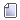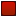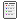###Author Topic: Call-By-Sharing  (Read 1666 times)

####Hooman

• Hero Member
••• Posts: 4948##### Call-By-Sharing
« on: October 07, 2017, 10:43:10 PM »
I came across a somewhat less common programming term: Call-By-Sharing

One characterization is:
Call-by-value, but object variables are understood to contain a reference to the actual object.

If you mutate a passed object, the change is seen by the caller. If you re-assign a value to a passed variable, it is not seen by the caller.

Python examples from the Wikipedia article:
Mutation is seen by caller:
Code: [Select]
``def f(l):    l.append(1)m = []f(m)print(m)  # => ``

Re-assignment is not seen by caller:
Code: [Select]
``def f(l):    l = m = []f(m)print(m)  # => []``

Applies to:
Quote
Python, Iota, Java (for object references), Ruby, JavaScript, Scheme, OCaml, AppleScript, and many others

Another characterization:
Primitive values use pass-by-value, while object variables use pass-by-reference.

Despite the name, for C++, this is more like pointer syntax than reference syntax. If you assigned to a reference (after the initial assignment that sets the address/object identity), it will copy over the original object. If you assign to a pointer, the pointer now holds a new distinct object, and the old object continues to exist in memory.
Code: [Select]
``#include <iostream>void f(int a, int& b, int* c, int* d){  a = 5;  // Assignment of value  b = 6;  // Assignment of reference (overwrites/mutates original object)  *c = 7; // Assignment through a reference (mutating original through a reference)  d = new int{8}; // Assignment of pointer (new object identity, separate from old)}int main(){  int a = 1;  int b = 2;  int c = 3;  int d = 4;  f(a, b, &c, &d);  std::cout << a << b << c << d << std::endl;  // => 1674}``

Edit:
There is also this nicely answered question on StackOverflow for JavaScript:
Is JavaScript a Pass-By-Reference or Pass-By-Value Language?
« Last Edit: October 07, 2017, 11:10:42 PM by Hooman »